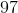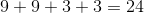# SSAT Elementary Level Math : How to find the perimeter of a rectangle

## Example Questions

← Previous 1 3 4 5 6 7 8 9 15 16

### Example Question #431 : Quadrilaterals

If Adam draws a rectangle with a long side of 6 cm and a short side of 5 cm, what is the perimeter?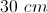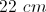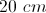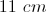Explanation:

To find the perimeter of a rectangle, add all four sides: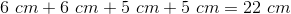### Example Question #432 : Quadrilaterals

One rectangular lane in a swimming pool isfeet wide andfeet long. What is the area of the lane?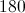square feet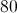square feet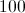square feet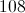square feet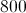square feetsquare feet

Explanation:

Area is calculated for a rectangle as width multiplied by length. In this case,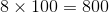square feet.

### Example Question #433 : Quadrilaterals

Which of the lists below could represent the side lengths of a rectangle?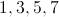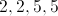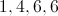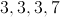Explanation:

Rectangles have two pairs of equal sides.

### Example Question #1 : How To Find The Perimeter Of A Rectangle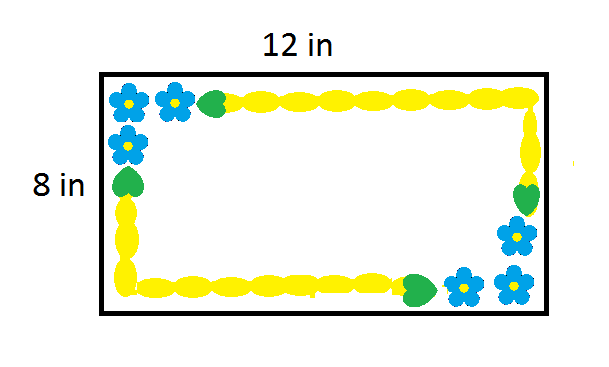A rectangular birthday cake (shown above) measures 8 inches by 12 inches. What is the perimeter of the cake?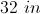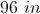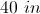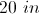Explanation: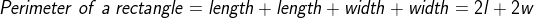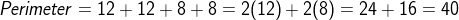### Example Question #442 : Quadrilaterals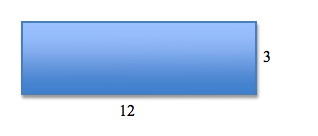What is the perimeter of the above rectangle?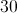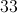Explanation:

To find the perimeter of this rectangle, add all four sides together. Even though we are not given the length of all four sides, we know that opposite sides are always equal.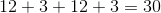### Example Question #443 : Quadrilaterals

A rectangular table can seatpeople on each of its longer sides andpeople on each of its shorter sides.

How many people are at the table when all the seats are filled?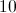people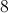people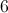peoplepeoplepeoplepeople

Explanation:

Since a rectangle has two pairs of equal-length sides, multiply the number of people that can sit on each side by, then add these numbers together to find the total number of people that can sit at the table.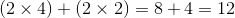people

### Example Question #444 : Quadrilaterals

If Elijah draws a rectangle with a long side of 9 cm and a short side of 3 cm, what is the perimeter of the rectangle?

28 cm

32 cm

27 cm

24 cm

24 cm

Explanation:

To find the perimeter of a rectangle, add all four sides: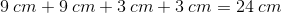### Example Question #445 : Quadrilaterals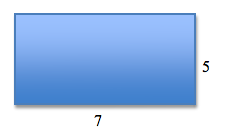What is the perimeter of the above rectangle?Explanation:

To find the perimeter of this rectangle, add all four sides together. Even though we are not given the length of all four sides, we know that opposite sides of a rectangle are always equal!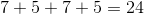### Example Question #446 : Quadrilaterals

What is the PERIMETER of the rectangle in the image?

80 cm

90 cm

40 cm

40 cm

300 cm

80 cm

Explanation:

Because the problem asks you to find the perimeter, you are looking for the total distance around the outside of the shape. The problem also tells you that the figure is a rectangle, so you know that all the sides are 10 cm and 30 cm. Adding all four sides gives the perimeter: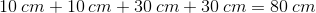### Example Question #447 : Quadrilaterals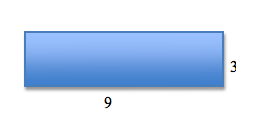What is the perimeter of the rectangle?